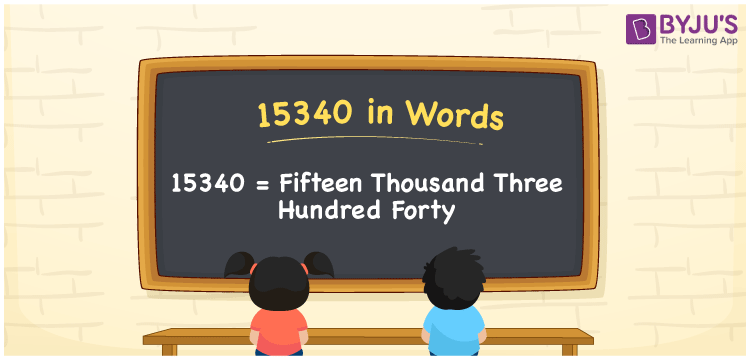# 15340 in Words

We can write 15340 in words as Fifteen Thousand Three Hundred Forty. It is possible to effortlessly convert the number 15340 into words using a place value chart. However, we use the word form of the number 15340 to read or express a certain amount, measure or quantity. For instance, to read the amount Rs. 15340, we use the words “Fifteen Thousand Three Hundred Forty Rupees”.

 15340 in words Fifteen Thousand Three Hundred Forty Fifteen Thousand Three Hundred Forty in Numbers 15340

## 15340 in English Words

Generally, we use the English alphabet to express numbers in words. Therefore, we can spell 15340 in English as “Fifteen Thousand Three Hundred Forty”.## How to Write 15340 in Words?

Let’s understand how to convert 15340 into words using a place value chart. The below table depicts the place values for all five digits of the number 15340.

 Ten thousands Thousands Hundreds Tens Ones 1 5 3 4 0

Here, ones = 0, tens = 4, hundreds = 3, thousands = 5, ten thousands = 1

By expanding these digits based on their place values, we get;

1 × Ten thousand + 5 × Thousand + 3 × Hundred + 4 × Ten + 0 × One

= 1 × 10000 + 5 × 1000 + 3 × 100 + 4 × 10 + 0 × 1

= 10000 + 5000 + 300 + 40

= Ten Thousand + Five thousand + Three hundred + Forty

= Fifteen thousand + Three hundred forty

= Fifteen Thousand Three Hundred Forty

15340 is a natural number that is the successor of 15339 and predecessor of 15341.

15340 in words – Fifteen Thousand Three Hundred Forty

Is 15340 an odd number? – No

Is 15340 an even number? – Yes

Is 15340 a prime number? – No

Is 15340 a composite number? – Yes

Is 15340 a perfect square number? – No

Is 15340 a perfect cube number? – No

## Frequently Asked Questions on 15340 in Words

Q1

### How do you write 15340 in words?

Using English words, we can write 15340 as Fifteen Thousand Three Hundred Forty.
Q2

### How do you write Rs. 15340 in words on a cheque?

On a cheque, we can write Rs. 15340 in words as “Fifteen Thousand Three Hundred Forty rupees only”.
Q3

### Find 8500 + 6840. Write the answer in words.

8500 + 6840 = 15340 Thus, the value of 8500 + 6840 is Fifteen Thousand Three Hundred Forty.
Q4

### Is 15,340 a perfect square number?

No, 15,340 is not a perfect square number.
Q5

### Is 15,340 divisible by 10?

Yes, 15,340 is divisible by 10.